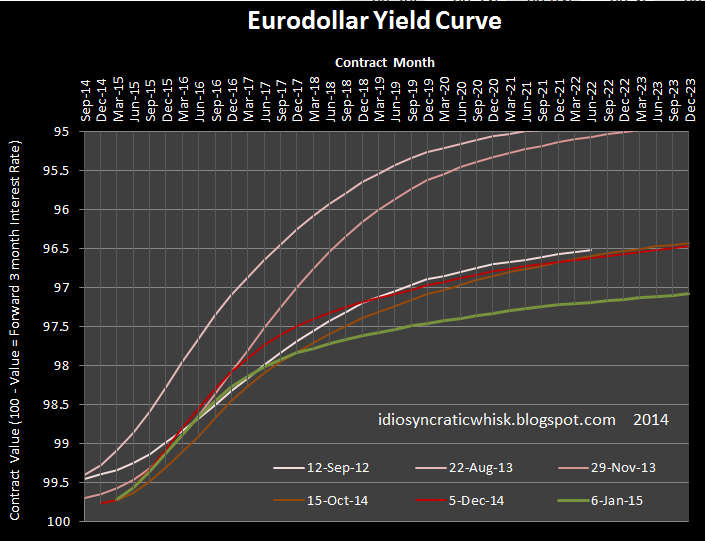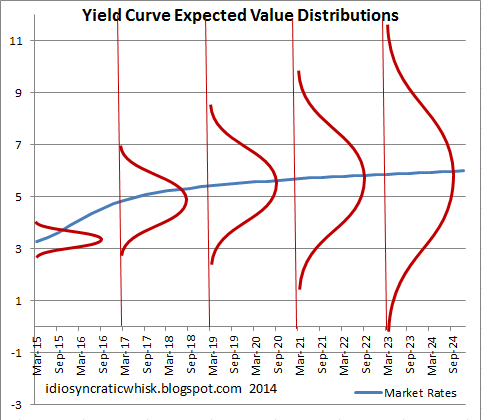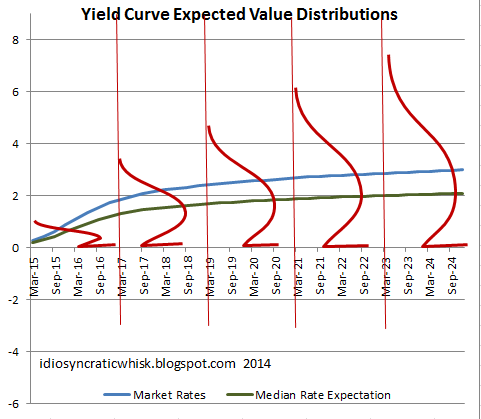## Wednesday, January 7, 2015

### Zero Lower Bound and Yield Curve Distortions

It's crunch time, and the signals are not comforting.Interest rates have taken a dive recently.  Declines this week appear to be from lower inflation premiums in the first 10 years of the yield curve and from both lower real and nominal rates at the long end of the curve.  The declines are not coming from a delay in rising rates in 2015.  The declines are coming entirely from lower rates at the long end and a lower slope during the rate recovery period.This first graph shows the expected date of the first rate hike and the expected subsequent rate of rate increases (from Eurodollar futures).  The expected date of the first hike has actually moved back in time recently (blue line, inverted right scale).  But the slope of rate hikes has dropped sharply down to 22 bps per quarter.

The next graph shows the Eurodollar curve at several points in time.  In Sept. 2012, when QE3 was just getting under way, short term rates were very close to where they are now.  Then, by the summer of 2013, both the date of the first hike and the rate of those hikes had taken bullish directions, with the first rate hike expected as early as 2014 and the slope hitting around 35 bps per quarter (which is still low relative to previous recoveries).  But, as QE3 has been tapered and then terminated, rates fell back to the previous level, and now long term rates are even lower than they had been after QE3 began.

The main difference (comparing the white line to the green line) is that the slope in late 2012 had been as low as 15 bps per quarter.  So, now, compared to late 2012, long term rates are lower, but the slope of the recovery period is somewhat higher.  My intuition here is that market participants are more confident that rates will rise at all than they were when QE3 began, but they now expect the long term posture of the Fed to be more hawkish.  But, I will address this further.  I think this may be a difficult thing to read.

My model of the expected date of the first hike, the uncertainty about that date, and the slope of the curve during subsequent hikes has been based on the notion of the short end of the yield curve at the zero lower bound (ZLB) as a sort of call option, where the ZLB is like the strike price, and the yield curve is the current "price" of forward rates, which includes an expectation of future rates and a premium created from the ZLB and the level of uncertainty about future rate movements.  A futures contract well into the future is like a call option far in-the-money.  Its price generally reflects the present value of future prices.  But, a futures contract near the expected date of the future rate hike includes a premium because the ZLB limits losses, just like a strike price does for a call option.

We are close enough to the expected rate hike that there isn't much "premium" curvature in the yield curve anymore.  And, at the very short end of the curve, Fed discretion can be as important as movements in natural rates.  So, I think the ZLB could be causing distortions now in the yield curve in a slightly different way.

We should think of the price of each forward interest rate as the price settled by the marginal investor in each contract.  The neutral final price that comes from a variety of different expectations.  So, each forward rate is the product, itself, of a distribution of expectations.

The recent decline in the slope of the yield curve has been roughly proportional to the decline in the expected long term interest rate.  So, compared to 4 months ago, I think there has been an increase in the expectation that we will, in fact, not escape the ZLB.  I have been thinking of this in terms of a bifurcated set of expectations, where some portion of the market expects rates to climb quickly to, say, 3% or 4%, and another portion expects us to remain at the ZLB or to leave it only temporarily.  Thinking of it that way, the ZLB traders would be pulling the yield curve down proportionately across the curve.But, I am wondering if this is the right way to think about it.  Here, I have graphed the yield curve as if short term rates were at 3%.  We might imagine that at each point along the curve, there are a range of relatively non-skewed expectations around the market rate.  As the yield curve moves out into the future, the uncertainty about the expected rate would increase, but the market rate would still be an unbiased reflection of a range of expectations.

But, if the rates are at the ZLB, then it is possible that the lower bound will affect the shape of expectations.  At very short term rates, this is basically how I have been modeling the convexity of the yield curve.  I had assumed that this factor was not important at the longer end of the yield curve.  But, as long rates have declined, the probability that there are some ZLB expectations at the long end of the curve seems undeniable.

If instead of thinking of it as consisting of a two-humped distribution, as I had above, what if we think of it as a normal distribution where the ZLB acts as a lower limit, much as a strike price does on a stock option?  This reverses the effect on forward market rates.Now, what we have is what would normally be the mean, median, and mode rate expectation.  But, the low end of the expectations distributions would be truncated by the ZLB.  We could presume that this would lessen the speculative weight these market participants would place on taking a position on lower rates, since the potential profit is limited by the ZLB.

If this is the basic shape of market expectations, then the ZLB would push the mean expected rates above the median expected rates - and presumably to some degree the market rates would also be pushed above the median expected rates.

In this scenario, the rate curve we are seeing now would actually be an overstatement of expected rates.  After walking through this, I think this is very likely to be the case.  If we take the scenario to the extreme, where expected future short term rates are expected to be at 0% indefinitely, we would clearly expect the long end of the curve to rise above zero, even if there was no maturity premium and we had a pure expectations context.  Because, there would be an option premium there, just as is with a stock option at the money or the recent short end of the yield curve.

The scale of this distortion would be a product of the level of uncertainty.  And this, ironically, might be good news.  The long end of the curve is about 3/4% lower than it was in September 2012 after the start of QE3.  While there are still some expectations of an enduring ZLB, there should be quite a bit less uncertainty about the near term economy than there was then.  GDP growth has remained steady.  Employment has been strengthening.  Commercial lending has continued to strengthen.  Households have continued to deleverage.

So, we should expect the tendency of the ZLB to push up the market rates at the long end of the yield curve to be weaker now than it was then.  Maybe the median expected long term rate level was, say, 2%, in September 2012 and is also at about that level now.  Maybe then the market rate was biased up by 1 3/4% and now it's only biased up by 1%, because there is a tighter range of expectations now.

Even if this is the case, the most recent drop in rates is probably the result of falling mean and median rate expectations and a higher probability of being stuck at the ZLB.

Also, the slope in the 2016 period is a monkey wrench in this hypothesis.  The expected date of the first rate increase has not changed.  If recent rate changes were a result of lower economic expectations, then I would have expected the date to move back in time.  It seems odd that the expected slope has declined while the expected date of the first increase has remained bullish.  This suggests the bifurcated expectations distribution I originally described.

The fact that the slope is higher now than in September 2012 also points to bifurcated expectations, since lower variance of expectations would have lowered the slope if expectations were normally distributed.

There is also the possibility that expectations about the way the Fed manages the rate of hikes in 2015-2017 has changed, and the changing slope simply reflects these changing expectations.  But, I'm already having trouble keeping this all straight without bringing in another vague and moving variable.

The ramifications for speculation are problematic here, because I would have been looking for a flat yield curve as a signal for declining short term rates.  This effect could mean that the next downturn begins when short term rates are near zero and the yield curve is distorted so that even if median expectations are for a flat yield curve, the market prices of forward rates could have a decent positive slope.

I hope that all of this becomes moot when we start to see mortgage credit flow and inflation expectations are re-invigorated.  But, there has been no sign of that happening yet.